# Rounding facts for kids

Kids Encyclopedia Facts

The word "rounding" for a numerical value means replacing it by another value that is approximately equal but has a shorter, simpler, or more explicit form. For example, US$23.74 could be rounded to US$24, or the fraction 312/937 could be rounded to 1/3, or the expression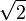$\sqrt{2}$ as 1.41.

Rounding is often done on purpose to obtain a value that is easier to write and handle than the original. It may be done also to indicate the accuracy of a computed number; for example, a quantity that was computed as 123,456 but is known to be accurate only to within a few hundred units is better stated as "about 123,500".

On the other hand, rounding can introduce some round-off error in the result. Rounding is almost unavoidable in many computations, especially when dividing two numbers in integer or fixed-point arithmetic; when computing mathematical functions such as square roots, logarithms, and sines; or when using a floating point representation with a fixed number of significant digits. In a sequence of calculations, these rounding errors generally accumulate, and in certain "ill-conditioned" cases, then they may make the result meaningless.

Accurate rounding of transcendental mathematical functions is difficult because the number of extra digits that need to be calculated to resolve whether to round up or down cannot be known in advance. This problem is known as "the table-maker's dilemma" (below).

Rounding has many similarities to the quantization that occurs when physical quantities must be encoded by numbers or digital signals.

## Types of rounding

Typical rounding problems are:

• approximating an irrational number by a fraction, e.g. π by 22/7;
• approximating a fraction with periodic decimal expansion by a finite decimal fraction, e.g. 5/3 by 1.6667;
• replacing a rational number by a fraction with smaller numerator and denominator, e.g. 3122/9417 by 1/3;
• replacing a fractional decimal number by one with fewer digits, e.g. 2.1784 dollars by 2.18 dollars;
• replacing a decimal integer by an integer with more trailing zeros, e.g. 23,217 people by 23,200 people; or, in general,
• replacing a value by a multiple of a specified amount, e.g. 27.2 seconds by 30 seconds (a multiple of 15).

## Rounding to a specified increment

The most common type of rounding is to round to an integer; or, more generally, to an integer multiple of some increment — such as rounding to whole tenths of seconds, hundredths of a dollar, to whole multiples of 1/2 or 1/8 inch, to whole dozens or thousands, etc..

In general, rounding a number x to a multiple of some specified increment m entails the following steps:

1. Divide x by m, let the result be y;
2. Round y to an integer value, call it q;
3. Multiply q by m to obtain the rounded value z.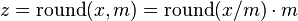$z = \mathrm{round}(x, m) = \mathrm{round}(x / m) \cdot m\,$

For example, rounding x = 2.1784 dollars to whole cents (i.e., to a multiple of 0.01) entails computing y = x/m = 2.1784/0.01 = 217.84, then rounding y to the integer q = 218, and finally computing z = q×m = 218×0.01 = 2.18.

When rounding to a predetermined number of significant digits, the increment m depends on the magnitude of the number to be rounded (or of the rounded result).

The increment m is normally a finite fraction in whatever numeral system that is used to represent the numbers. For display to humans, that usually means the decimal numeral system (that is, m is an integer times a power of 10, like 1/1000 or 25/100). For intermediate values stored in digital computers, it often means the binary numeral system (m is an integer times a power of 2).

The abstract single-argument "round()" function that returns an integer from an arbitrary real value has at least a dozen distinct concrete definitions presented in the rounding to integer section. The abstract two-argument "round()" function is formally defined here, but in many cases it is used with the implicit value m = 1 for the increment and then reduces to the equivalent abstract single-argument function, with also the same dozen distinct concrete definitions.

## Rounding to integer

The most basic form of rounding is to replace an arbitrary number by an integer. All the following rounding modes are concrete implementations of the abstract single-argument "round()" function presented and used in the previous sections.

There are many ways of rounding a number y to an integer q. The most common ones are

• round down (or take the floor, or round towards minus infinity): q is the largest integer that does not exceed y.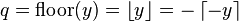$q = \mathrm{floor}(y) = \left\lfloor y \right\rfloor = -\left\lceil -y \right\rceil\,$
• round up (or take the ceiling, or round towards plus infinity): q is the smallest integer that is not less than y.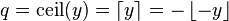$q = \mathrm{ceil}(y) = \left\lceil y \right\rceil = -\left\lfloor -y \right\rfloor\,$
• round towards zero (or truncate, or round away from infinity): q is the integer part of y, without its fraction digits.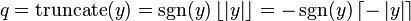$q = \mathrm{truncate}(y) = \sgn(y) \left\lfloor \left| y \right| \right\rfloor = -\sgn(y) \left\lceil -\left| y \right| \right\rceil\,$
• round away from zero (or round towards infinity): if y is an integer, q is y; else q is the integer that is closest to 0 and is such that y is between 0 and q.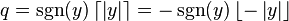$q = \sgn(y) \left\lceil \left| y \right| \right\rceil = -\sgn(y) \left\lfloor -\left| y \right| \right\rfloor\,$
• round to nearest: q is the integer that is closest to y (see below for tie-breaking rules).

The first four methods are called directed rounding, as the displacements from the original number y to the rounded value q are all directed towards or away from the same limiting value (0, +∞, or −∞).

If y is positive, round-down is the same as round-towards-zero, and round-up is the same as round-away-from-zero. If y is negative, round-down is the same as round-away-from-zero, and round-up is the same as round-towards-zero. In any case, if y is integer, q is just y. The following table illustrates these rounding methods:

y round
down
(towards −∞)
round
up
(towards +∞)
round
towards
zero
round
away from
zero
round
to
nearest
+23.67 +23 +24 +23 +24 +24
+23.50 +23 +24 +23 +24 +23 or +24
+23.35 +23 +24 +23 +24 +23
+23.00 +23 +23 +23 +23 +23
0 0 0 0 0 0
−23.00 −23 −23 −23 −23 −23
−23.35 −24 −23 −23 −24 −23
−23.50 −24 −23 −23 −24 −23 or −24
−23.67 −24 −23 −23 −24 −24

Where many calculations are done in sequence, the choice of rounding method can have a very significant effect on the result. A famous instance involved a new index set up by the Vancouver Stock Exchange in 1982. It was initially set at 1000.000, and after 22 months had fallen to about 520 — whereas stock prices had generally increased in the period. The problem was caused by the index being recalculated thousands of times daily, and always being rounded down to 3 decimal places, in such a way that the rounding errors accumulated. Recalculating with better rounding gave an index value of 1098.892 at the end of the same period.

## Tie-breaking

Rounding a number y to the nearest integer requires some tie-breaking rule for those cases when y is exactly half-way between two integers — that is, when the fraction part of y is exactly 0.5.

### Round half up

The following tie-breaking rule, called round half up (or round half towards plus infinity), is widely used in many disciplines. That is, half-way values y are always rounded up.

• If the fraction of y is exactly 0.5, then q = y + 0.5.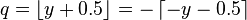$q = \left\lfloor y + 0.5 \right\rfloor = -\left\lceil -y - 0.5 \right\rceil\,$

For example, by this rule the value 23.5 gets rounded to 24, but −23.5 gets rounded to −23.

This is one of two rules generally taught in US elementary mathematics classes.

If it were not for the 0.5 fractions, the roundoff errors introduced by the round to nearest method would be quite symmetric: for every fraction that gets rounded up (such as 0.268), there is a complementary fraction (namely, 0.732) that gets rounded down, by the same amount. When rounding a large set of numbers with random fractional parts, these rounding errors would statistically compensate each other, and the expected (average) value of the rounded numbers would be equal to the expected value of the original numbers.

However, the round half up tie-breaking rule is not symmetric, as the fractions that are exactly 0.5 always get rounded up. This asymmetry introduces a positive bias in the roundoff errors. For example, if the fraction of y consists of three random decimal digits, then the expected value of q will be 0.0005 higher than the expected value of y. For this reason, round-to-nearest with the round half up rule is also (ambiguously) known as asymmetric rounding.

One reason for rounding up at 0.5 is that only one digit need be examined. When seeing 17.50000..., for example, the first three figures, 17.5, determines that the figure would be rounded up to 18. If the opposite rule were used (round half down), then all the zero decimal places would need to be examined to determine if the value is exactly 17.5.

### Round half down

One may also use round half down (or round half towards minus infinity) as opposed to the more common round half up (the round half up method is a common convention, but is nothing more than a convention).

• If the fraction of y is exactly 0.5, then q = y − 0.5.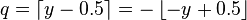$q = \left\lceil y - 0.5 \right\rceil = -\left\lfloor -y + 0.5 \right\rfloor\,$

For example, 23.5 gets rounded to 23, and −23.5 gets rounded to −24.

The round half down tie-breaking rule is not symmetric, as the fractions that are exactly 0.5 always get rounded down. This asymmetry introduces a negative bias in the roundoff errors. For example, if the fraction of y consists of three random decimal digits, then the expected value of q will be 0.0005 lower than the expected value of y. For this reason, round-to-nearest with the round half down rule is also (ambiguously) known as asymmetric rounding.

### Round half away from zero

The other tie-breaking method commonly taught and used is the round half away from zero (or round half towards infinity), namely:

• If the fraction of y is exactly 0.5, then q = y + 0.5 if y is positive, and q = y − 0.5 if y is negative.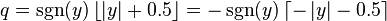$q = \sgn(y) \left\lfloor \left| y \right| + 0.5 \right\rfloor = -\sgn(y) \left\lceil -\left| y \right| - 0.5 \right\rceil \,$

For example, 23.5 gets rounded to 24, and −23.5 gets rounded to −24.

This method treats positive and negative values symmetrically, and therefore is free of overall bias if the original numbers are positive or negative with equal probability. However, this rule will still introduce a positive bias for positive numbers, and a negative bias for the negative ones.

It is often used for currency conversions and price roundings (when the amount is first converted into the smallest significant subdivision of the currency, such as cents of a euro) as it is easy to explain by just considering the first fractional digit, independently of supplementary precision digits or sign of the amount (for strict equivalence between the paying and recipient of the amount).

### Round half towards zero

One may also round half towards zero (or round half away from infinity) as opposed to the more common round half away from zero (the round half away from zero method is a common convention, but is nothing more than a convention).

• If the fraction of y is exactly 0.5, then q = y − 0.5 if y is positive, and q = y + 0.5 if y is negative.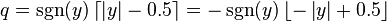$q = \sgn(y) \left\lceil \left| y \right| - 0.5 \right\rceil = -\sgn(y) \left\lfloor -\left| y \right| + 0.5 \right\rfloor \,$

For example, 23.5 gets rounded to 23, and −23.5 gets rounded to −23.

This method also treats positive and negative values symmetrically, and therefore is free of overall bias if the original numbers are positive or negative with equal probability. However, this rule will still introduce a negative bias for positive numbers, and a positive bias for the negative ones.

### Round half to even

A tie-breaking rule that is even less biased is round half to even, namely

• If the fraction of y is 0.5, then q is the even integer nearest to y.

Thus, for example, +23.5 becomes +24, +22.5 becomes +22, −22.5 becomes −22, and −23.5 becomes −24.

This method also treats positive and negative values symmetrically, and therefore is free of overall bias if the original numbers are positive or negative with equal probability. In addition, for most reasonable distributions of y values, the expected (average) value of the rounded numbers is essentially the same as that of the original numbers, even if the latter are all positive (or all negative). However, this rule will still introduce a positive bias for even numbers (including zero), and a negative bias for the odd ones.

This variant of the round-to-nearest method is also called unbiased rounding (ambiguously, and a bit abusively), convergent rounding, statistician's rounding, Dutch rounding, Gaussian rounding, or bankers' rounding. This is widely used in bookkeeping.

This is the default rounding mode used in IEEE 754 computing functions and operators.

### Round half to odd

Another tie-breaking rule that is very similar to round half to even, namely

• If the fraction of y is 0.5, then q is the odd integer nearest to y.

Thus, for example, +22.5 becomes +23, +21.5 becomes +21, −21.5 becomes −21, and −22.5 becomes −23.

This method also treats positive and negative values symmetrically, and therefore is free of overall bias if the original numbers are positive or negative with equal probability. In addition, for most reasonable distributions of y values, the expected (average) value of the rounded numbers is essentially the same as that of the original numbers, even if the latter are all positive (or all negative). However, this rule will still introduce a negative bias for even numbers (including zero), and a positive bias for the odd ones.

This variant is almost never used in most computations, except in situations where one wants to avoid rounding 0.5 or −0.5 to zero, or to avoid increasing the scale of numbers represented as floating point (with limited ranges for the scaling exponent), so that a non infinite number would round to infinite, or that a small denormal value would round to a normal non-zero value (these could occur with the round half to even mode). Effectively, this mode prefers preserving the existing scale of tie numbers, avoiding out of range results when possible.

### Stochastic rounding

Another unbiased tie-breaking method is stochastic rounding:

• If the fractional part of y is .5, choose q randomly among y + 0.5 and y − 0.5, with equal probability.

Like round-half-to-even, this rule is essentially free of overall bias; but it is also fair among even and odd q values. On the other hand, it introduces a random component into the result; performing the same computation twice on the same data may yield two different results. Also, it is open to unconscious bias if humans (rather than computers or devices of chance) are "randomly" deciding in which direction to round.

### Alternating tie-breaking

One method, more obscure than most, is round half alternatingly.

• If the fractional part is 0.5, alternate round up and round down: for the first occurrence of a 0.5 fractional part, round up; for the second occurrence, round down; so on so forth.

This suppresses the random component of the result, if occurrences of 0.5 fractional parts can be effectively numbered. But it can still introduce a positive or negative bias according to the direction of rounding assigned to the first occurrence, if the total number of occurrences is odd.

## Simple dithering

In some contexts, all the rounding methods above may be unsatisfactory. For example, suppose that y is an accurate measurement of an audio signal, which is being rounded to an integer q in order to reduce the storage or transmission costs. If y changes slowly with time, any of the rounding method above will result in q being completely constant for long intervals, separated by sudden jumps of ±1. When the q signal is played back, these steps will be heard as a very disagreeable noise, and any variations of the original signal between two integer values will be completely lost.

One way to avoid this problem is to round each value y upwards with probability equal to its fraction, and round it downwards with the complement of that probability. For example, the number 23.17 would be rounded up to 24 with probability 0.17, and down to 23 with probability 1 - 0.17 = 0.83. (This is equivalent to rounding down y + s, where s is a random number uniformly distributed between 0 and 1.) With this special rounding, known as dithering, the sudden steps get replaced by a less objectionable noise, and even small variations in the original signal will be preserved to some extent. Like the stochastic approach to tie-breaking, dithering has no bias: if all fraction values are equally likely, rounding up by a certain amount is as likely as rounding down by that same amount; and the same is true for the sum of several rounded numbers. On the other hand, dithering introduces a random component in the result, much greater than that of stochastic tie-breaking.

More precisely, the roundoff error for each dithered number will be a uniformly distributed random variable with mean value of zero, but with a standard deviation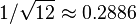$1 / \sqrt{12} \approx 0.2886$, which is better than the 1/2 standard deviation with the simple predictive methods, but slightly higher than with the simpler stochastic method. However, the sum of n rounded numbers will be a random variable with expected error zero, but with standard deviation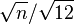$\sqrt{n} / \sqrt{12}$ (the total remaining noise) which diverges semi-quadratically and may become easily perceptible, even if the standard deviation of the roundoff error per sample will be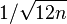$1 / \sqrt{12 n}$ which slowly converges semi-quadratically to zero. So, this random distribution may still be too high for some applications that are rounding a lot of data.

## Multidimensional dithering

This variant of the simple dithering method still rounds values with probability equal to its fraction. However, instead of using a random distribution for rounding isolated samples, the roundoff error occurring at each rounded sample is totalled for the next surrounding elements to sample or compute; this accumulated value is then added to the value of these next sampled or computed values to round, so that the modified values will take into account this difference using a predictive model (such as Floyd–Steinberg dithering).

The modified values are then rounded with any one of the above rounding methods, the best ones being with stochastic or dithering methods: in this last case, the sum of n rounded numbers will still be a random variable with expected error zero but with an excellent constant standard deviation of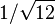$1 / \sqrt{12}$, instead of diverging semi-quadratically when dithering isolated samples; and the overall average roundoff error deviation per rounded sample will be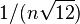$1 / (n \sqrt{12})$ that will converge hyperbolically to zero, faster than with the semi-hyperbolic convergence when dithering isolated samples.

In practice, when rounding large sets of sampled data (such as audio, image and video rendering), the accumulation of roundoff errors is most frequently used with a simple predictive rounding of the modified values (such as rounding towards zero), because it will still preserve the hyperbolic convergence towards zero of the overall mean roundoff error bias and of its standard deviation. This enhancement is frequently used in image and audio processing (notably for accurate rescaling and antialiasing operations, where the simple probabilistic dithering of isolated values may still produce perceptible noise, sometimes even worse than the moiré effects occurring with simple non-probabilistic rounding methods applied to isolated samples).

The effective propagation of accumulated roundoff errors may depend on the discrete dimension of the sampled data to round: when sampling bidimensional images, including colored images (that add the discrete dimension of color planes), or tridimensional videos (that add a discrete time dimension), or on polyphonic audio data (using time and channel discrete dimensions), it may still be preferable to propagate this error into a preferred direction, or equally into several orthogonal dimensions, such as vertically vs. horizontally for bidimensional images, or into parallel color channels at the same position and/or timestamp, and depending on other properties of these orthogonal discrete dimensions (according to a perception model). In those cases, several roundoff error accumulators may be used (at least one for each discrete dimension), or a (n-1)-dimension vector (or matrix) of accumulators.

In some of these cases, the discrete dimensions of the data to sample and round may be treated non orthogonally: for example, when working with colored images, the trichromatic color planes data in each physical dimension (height, width and optionally time) could be remapped using a perceptive color model, so that the roundoff error accumulators will be designed to preserve lightness with a higher probability than hue or saturation, instead of propagating errors into each orthogonal color plane independently; and in stereophonic audio data the two rounded data channels (left and right) may be rounded together to preserve their mean value in priority to their effective difference that will absorb most of the remaining roundoff errors, in a balanced way around zero.

## Rounding to simple fractions

In some contexts it is desirable to round a given number x to a "neat" fraction — that is, the nearest fraction z = m/n whose numerator m and denominator n do not exceed a given maximum. This problem is fairly distinct from that of rounding a value to a fixed number of decimal or binary digits, or to a multiple of a given unit m. This problem is related to Farey sequences, the Stern-Brocot tree, and continued fractions.

## Scaled rounding

This type of rounding, which is also named rounding to a logarithmic scale, is a variant of Rounding to a specified increment but with an increment that is modified depending on the scale and magnitude of the result. Concretely, the intent is to limit the number of significant digits, rounding the value so that non-significant digits will be dropped. This type of rounding occurs implicitly with numbers computed with floating-point values with limited precision (such as IEEE-754 float and double types), but it may be used more generally to round any real values with any positive number of significant digits and any strictly positive real base.

For example it can be used in engineering graphics for representing data with a logarithmic scale with variable steps (for example wave lengths, whose base is not necessarily an integer measure), or in statistical data to define classes of real values within intervals of exponentially growing widths (but the most common use is with integer bases such as 10 or 2).

This type of rounding is based on a logarithmic scale defined by a fixed non-zero real scaling factor s (in most frequent cases this factor is s=1) and a fixed positive base b>1 (not necessarily an integer and most often different from the scaling factor), and a fixed integer number n>0 of significant digits in that base (which will determine the value of the increment to use for rounding, along with the computed effective scale of the rounded number).

The primary argument number (as well as the resulting rounded number) is first represented in exponential notation x = s·a·m·bc, such that the sign s is either +1 or −1, the absolute mantissa a is restricted to the half-open positive interval [1/b,1), and the exponent c is any (positive or negative) integer. In that representation, all significant digits are in the fractional part of the absolute mantissa whose integer part is always zero.

If the source number (or rounded number) is 0, the absolute mantisssa a is defined as 0, the exponent c is fixed to an arbitrary value (0 in most conventions, but some floating-point representations cannot use a null absolute mantissa but reserve a specific maximum negative value for the exponent c to represent the number 0 itself), and the sign s may be arbitrarily chosen between −1 or +1 (it is generally set to +1 for simple zero, or it is set to the same sign as the argument in the rounded value if the number representation allows to differentiate positive and negative zeroes, even if they finally represent the same numeric value 0).

A scaled exponential representation as x = a·s·bc may also be used equivalently, with a signed mantissa a either equal to zero or within one of the two half-open intervals (−1,−1/b] and [+1/b,+1), and this will be the case in the algorithm below.

The steps to compute this scaled rounding are generally similar to the following:

1. if x equals zero, simply return x; otherwise:
2. convert x into the scaled exponential representation, with a signed mantissa: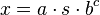$x = a \cdot s \cdot b^c\,$
1. let x’ be the unscaled value of x, by dividing it by the scaling factor s: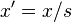$x' = x / s\,$;
2. let the scaling exponent c be one plus the base-b logarithm of the absolute value of x’, rounded down to an integer (towards minus infinity):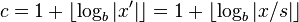$c = 1 + \left\lfloor \log_b \left| x' \right| \right\rfloor = 1 + \left\lfloor \log_b \left| x / s \right| \right\rfloor\,$;
3. let the signed mantissa a be product of x’ divided by b to the power c: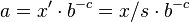$a = x' \cdot b^{-c} = x / s \cdot b^{-c}\,$
3. compute the rounded value in this representation:
1. let c’ be the initial scaling exponent c of x’: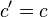$c' = c\,$
2. let m be the increment for rounding the mantissa a according to the number of significant digits to keep: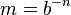$m = b^{-n}\,$
3. let a’ be the signed mantissa a rounded according to this increment m and the selected rounding mode: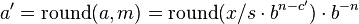$a' = \mathrm{round}(a, m) = \mathrm{round}(x / s \cdot b^{n-c'}) \cdot b^{-n}\,$
4. if the absolute value of a’ is not lower than b, then decrement n (multiply the increment m by b), increment the scaling exponent c’, divide the signed mantissa a by b, and restart the rounding of the new signed mantissa a into a’ with the same formula; this step may be avoided only if the abtract "round()" function is always rounding a towards 0 (i.e. when it is a simple truncation), but is necessary if it may be rounding a towards infinity, because the rounded mantissa may have a higher scaling exponent in this case, leaving an extra digit of precision.
4. return the rounded value: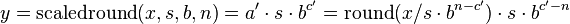$y = \mathrm{scaledround}(x, s, b, n) = a' \cdot s \cdot b^{c'} = \mathrm{round}(x / s \cdot b^{n-c'}) \cdot s \cdot b^{c'-n}\,$.

For the abstract "round()" function, this type of rounding can use any one of the rounding to integer modes described more completely in the next section, but it is most frequently the round to nearest mode (with tie-breaking rules also described more completely below).

For example:

• the scaled rounding of 1.234 with scaling factor 1 in base 10 and 3 significant digits (maximum relative precision=1/1000), when using any round to nearest mode, will return 1.23;
• similar scaled rounding of 1.236 will return 1.24;
• similar scaled rounding of 21.236 will return 21.2;
• similar scaled rounding of 321.236 will return 321;
• the scaled rounding of 1.234 scaling factor 1 in base 10 and 3 significant digits (maximum relative precision=1/1000), when using the round down mode, will return 1.23;
• similar scaled rounding of 1.236 will also return 1.23;
• the scaled rounding of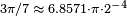$3\pi/7 \; \approx \; 6.8571 \cdot \pi \cdot 2^{-4}$ with scaling factor$\pi$ in base 2 and 3 significant digits (maximum relative precision=1/8), when using the round down mode, will return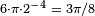$6 \cdot \pi \cdot 2^{-4} \; = \; 3\pi/8$;
• similar scaled rounding of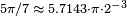$5\pi/7 \; \approx \; 5.7143 \cdot \pi \cdot 2^{-3}$ will return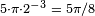$5 \cdot \pi \cdot 2^{-3} \; = \; 5\pi/8$;
• similar scaled rounding of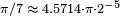$\pi/7 \; \approx \; 4.5714 \cdot \pi \cdot 2^{-5}$ will return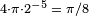$4 \cdot \pi \cdot 2^{-5} \; = \; \pi/8$.
• similar scaled rounding of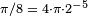$\pi/8 \; = \; 4 \cdot \pi \cdot 2^{-5}$ will also return$4 \cdot \pi \cdot 2^{-5} \; = \; \pi/8$.
• similar scaled rounding of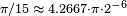$\pi/15 \; \approx \; 4.2667 \cdot \pi \cdot 2^{-6}$ will return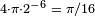$4 \cdot \pi \cdot 2^{-6} \; = \; \pi/16$.

## Round to available value

Finished lumber, writing paper, capacitors, and many other products are usually sold in only a few standard sizes.

Many design procedures describe how to calculate an approximate value, and then "round" to some standard size using phrases such as "round down to nearest standard value", "round up to nearest standard value", or "round to nearest standard value".

When a set of preferred values is equally spaced on a logarithmic scale, Choosing the closest preferred value to any given value can be seen as a kind of scaled rounding. Such "rounded" values can be directly calculated.

## Floating-point rounding

In floating-point arithmetic, rounding aims to turn a given value x into a value z with a specified number of significant digits. In other words, z should be a multiple of a number m that depends on the magnitude of z. The number m is a power of the base (usually 2 or 10) of the floating-point form.

Apart from this detail, all the variants of rounding discussed above apply to the rounding of floating-point numbers as well. The algorithm for such rounding is presented in the Scaled rounding section above, but with a constant scaling factor s=1, and an integer base b>1.

For results where the rounded result would overflow the result for a directed rounding is either the appropriate signed infinity, or the highest representable positive finite number (or the lowest representable negative finite number if x is negative), depending on the direction of rounding. The result of an overflow for the usual case of round to even is always the appropriate infinity.

In addition, if the rounded result would underflow, i.e. if the exponent would exceed the lowest representable integer value, the effective result may be either zero (possibly signed if the representation can maintain a distinction of signs for zeroes), or the smallest representable positive finite number (or the highest representable negative finite number if x is negative), possibly a denormal positive or negative number (if the mantissa is storing all its significant digits, in which case the most significant digit may still be stored in a lower position by setting the highest stored digits to zero, and this stored mantissa does not drop the most significant digit, something that is possible when base b=2 because the most significant digit is always 1 in that base), depending on the direction of rounding. The result of an underflow for the usual case of round to even is always the appropriate zero.

## Double rounding

Rounding a number twice in succession to different precisions, with the latter precision being coarser, is not guaranteed to give the same result as rounding once to the final precision except in the case of directed rounding. For instance rounding 9.46 to one decimal gives 9.5, and then 10 when rounding to integer using rounding half to even, but would give 9 when rounded to integer directly.

Some computer languages and the IEEE 754-2008 standard dictate that in straightforward calculations, the result should not be rounded twice. This has been a particular problem with Java as it is designed to be run identically on different machines, special programming tricks have had to be used to achieve this with x87 floating point. The Java language was changed to allow different results where the difference does not matter and require a "strictfp" qualifier to be used when the results have to conform accurately.

## Exact computation with rounded arithmetic

It is possible to use rounded arithmetic to evaluate the exact value of a function with a discrete domain and range. For example, if we know that an integer n is a perfect square, we can compute its square root by converting n to a floating-point value x, computing the approximate square root y of x with floating point, and then rounding y to the nearest integer q. If n is not too big, the floating-point roundoff error in y will be less than 0.5, so the rounded value q will be the exact square root of n. In most modern computers, this method may be much faster than computing the square root of n by an all-integer algorithm.

## The table-maker's dilemma

William Kahan coined the term "The Table-Maker's Dilemma" for the unknown cost of rounding transcendental functions:

"Nobody knows how much it would cost to compute y^w correctly rounded for every two floating-point arguments at which it does not over/underflow. Instead, reputable math libraries compute elementary transcendental functions mostly within slightly more than half an ulp and almost always well within one ulp. Why can't Y^W be rounded within half an ulp like SQRT? Because nobody knows how much computation it would cost... No general way exists to predict how many extra digits will have to be carried to compute a transcendental expression and round it correctly to some preassigned number of digits. Even the fact (if true) that a finite number of extra digits will ultimately suffice may be a deep theorem."

The IEEE floating point standard guarantees that add, subtract, multiply, divide, square root, and floating point remainder will give the correctly rounded result of the infinite precision operation. However, no such guarantee is given for more complex functions and they are typically only accurate to within the last bit at best.

Using the Gelfond–Schneider theorem and Lindemann–Weierstrass theorem, many of the standard elementary functions can be proved to return transcendental results when given rational non-zero arguments; therefore it is always possible to correctly round such functions. However determining a limit for a given precision on how accurate results needs to be computed before a correctly rounded result can be guaranteed may demand a lot of computation time.

There are some packages around now that offer full accuracy. The MPFR package gives correctly rounded arbitrary precision results. IBM has written a package for fast and accurate IEEE elementary functions and in the future the standard libraries may offer such precision.

It is possible to devise well-defined computable numbers which it may never be possible to correctly round no matter how many digits are calculated. For instance, if Goldbach's conjecture is true but unprovable, then it is impossible to correctly round down 0.5 + 10-n where n is the first even number greater than 4 which is not the sum of two primes, or 0.5 if there is no such number. This can however be approximated to any given precision even if the conjecture is unprovable.

## History

The concept of rounding is very old, perhaps older even than the concept of division. Some ancient clay tablets found in Mesopotamia contain tables with rounded values of reciprocals and square roots in base 60. Rounded approximations to π, the length of the year, and the length of the month are also ancient.

The Round-to-even method has served as the ASTM (E-29) standard since 1940. The origin of the terms unbiased rounding and statistician's rounding are fairly self-explanatory. In the 1906 4th edition of Probability and Theory of Errors Robert Simpson Woodward called this "the computer's rule" indicating that it was then in common use by human computers who calculated mathematical tables. Churchill Eisenhart's 1947 paper "Effects of Rounding or Grouping Data" (in Selected Techniques of Statistical Analysis, McGrawHill, 1947, Eisenhart, Hastay, and Wallis, editors) indicated that the practice was already "well established" in data analysis.

The origin of the term "bankers' rounding" remains more obscure. If this rounding method was ever a standard in banking, the evidence has proved extremely difficult to find. To the contrary, section 2 of the European Commission report The Introduction of the Euro and the Rounding of Currency Amounts suggests that there had previously been no standard approach to rounding in banking; and it specifies that "half-way" amounts should be rounded up.

Until the 1980s, the rounding method used in floating-point computer arithmetic was usually fixed by the hardware, poorly documented, inconsistent, and different for each brand and model of computer. This situation changed after the IEEE 754 floating point standard was adopted by most computer manufacturers. The standard allows the user to choose among several rounding modes, and in each case, specifies precisely how the results should be rounded. These features made numerical computations more predictable and machine-independent, and made possible the efficient and consistent implementation of interval arithmetic.

## Rounding functions in programming languages

Most programming languages provide functions or special syntax to round fractional numbers in various ways. The earliest numeric languages, such as FORTRAN and C, would provide only one method, usually truncation (towards zero). This default method could be implied in certain contexts, such as when assigning a fractional number to an integer variable, or using a fractional number as an index of an array. Other kinds of rounding had to be programmed explicitly; for example, rounding a positive number to the nearest integer could be implemented by adding 0.5 and truncating.

In the last decades, however, the syntax and/or the standard libraries of most languages have commonly provided at least the four basic rounding functions (up/ceiling, down/floor, to nearest, and towards zero). The tie-breaking method may vary depending the language and version, and/or may be selectable by the programmer. Several languages follow the lead of the IEEE-754 floating-point standard, and define these functions as taking a double precision float argument and returning the result of the same type, which then may be converted to an integer if necessary. Since the IEEE double precision format has 52 fraction bits, this approach may avoid spurious overflows in languages have 32-bit integers. Some languages, such as PHP, provide functions that round a value to a specified number of decimal digits, e.g. from 4321.5678 to 4321.57 or 4300. In addition, many languages provide a "printf" or similar string formatting function, which allows one to convert a fractional number to a string, rounded to a user-specified number of decimal places (the precision). On the other hand, truncation (round to zero) is still the default rounding method used by many languages, especially for the division of two integer values.

On the opposite, CSS and SVG do not define any specific maximum precision for numbers and measurements, that are treated and exposed in their Document Object Model and in their Interface-description-language interface as strings as if they had infinite precision, and do not discriminate between integers and floating point values; however, the implementations of these languages will typically convert these numbers into IEEE-754 double floating points before exposing the computed digits with a limited precision (notably within standard Javascript or ECMAScript interface bindings).

## Other rounding standards

Some disciplines or institutions have issued standards or directives for rounding.

### U.S. Weather Observations

In a guideline issued in mid-1966, the U.S. Office of the Federal Coordinator for Meteorology determined that weather data should be rounded to the nearest round number, with the "round half up" tie-breaking rule. For example, 1.5 rounded to integer should become 2, and −1.5 should become −1. Prior to that date, the tie-breaking rule was "round half away from zero".

### Negative zero in meteorology

Some meteorologists may write "-0" to indicate a temperature between 0.0 and -0.5 degrees (exclusive) that was rounded to integer. This notation is used when the negative sign is considered important, no matter how small is the magnitude; for example, when rounding temperatures in the Celsius scale, where below zero indicates freezing.

## Related pages

An introduction to different rounding algorithms that is accessible to a general audience but especially useful to those studying computer science and electronics.Rounding Facts for Kids. Kiddle Encyclopedia.